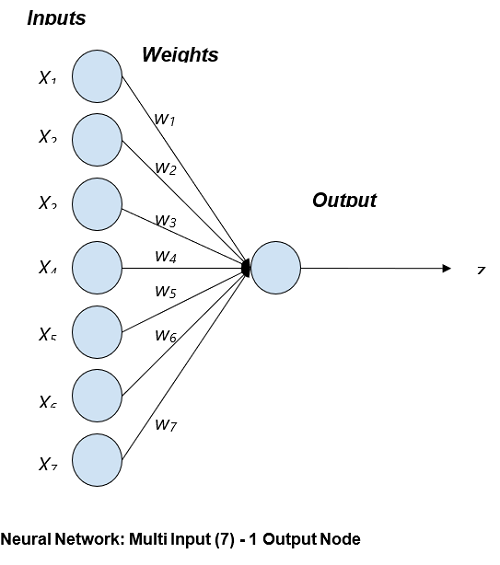# Uni - Layer Neural Network | Linear Algebra using Python

Linear Algebra using Python | Uni - Layer Neural Network: Here, we are going to learn about the uni - layer neural network and its implementation in Python.
Submitted by Anuj Singh, on June 03, 2020

A neural network is a powerful tool often utilized in Machine Learning because neural networks are fundamentally very mathematical. We will use our basics of Linear Algebra and NumPy to understand the foundation of Machine Learning using Neural Networks.

Our article is a showcase of the application of Linear Algebra and, Python provides a wide set of libraries that help to build our motivation of using Python for machine learning.

The figure is showing a neural network with multi-input and one output node.Input to the neural network is X1, X2,  X3……... Xn and their corresponding weights are w1, w2, w3………..wn respectively. The output z is a tangent hyperbolic function for decision making which has input as the sum of products of Input and Weight.

Mathematically,  z = tanh(∑ Xiwi)

Where tanh( ) is an tangent hyperbolic function (Refer article Linear Algebra | Tangent Hyperbolic Function) because it is one of the most used decision-making functions.

So for drawing this mathematical network in a python code by defining a function neural_network( X, W). Note: The tangent hyperbolic function takes input within the range of 0 to 1.

Input parameter(s): Vector X and W

Return: A value ranging between 0 and 1, as a prediction of the neural network based on the inputs.

Application:

1. Machine Learning
2. Computer Vision
3. Data Analysis
4. Fintech

## Python program for Uni - Layer Neural Network

```#Linear Algebra and Neural Network
#Linear Algebra Learning Sequence

import numpy as np

#Use of np.array() to define an Input Vector
inp = np.array([0.323, 0.432, 0.546, 0.44, 0.123, 0.78, 0.123])
print("The Vector A : ",inp)

#defining Weight Vector
weigh = np.array([0.3, 0.63, 0.99, 0.89, 0.50, 0.66, 0.123])
print("\nThe Vector B : ",weigh)

#defining a neural network for predicting an output value
def neural_network(inputs, weights):
wT = np.transpose(weights)
elpro = wT.dot(inputs)

#Tangent Hyperbolic Function for Decision Making
out = np.tanh(elpro)
return out

outputi = neural_network(inp,weigh)

#printing the expected output
print("\nExpected Output of the given Input data and their respective Weight : ", outputi)
```

Output:

```The Vector A :  [0.323 0.432 0.546 0.44  0.123 0.78  0.123]

The Vector B :  [0.3   0.63  0.99  0.89  0.5   0.66  0.123]

Expected Output of the given Input data and their respective Weight :  0.9556019596251646
```

Preparation

What's New

Top Interview Coding Problems/Challenges!